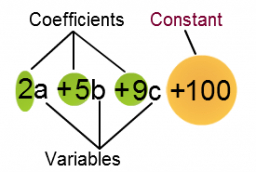# Expression 68814

Write the expression n, which is:
(a) Six greater than twice the expression y;

b) equal to the square root of the sum of the expression y and the number 3;

(c) three times less than four times the expression y.

a = 2y+6
b = sqrt(y+3)
c = 4y/3

### Step-by-step explanation:Did you find an error or inaccuracy? Feel free to write us. Thank you!